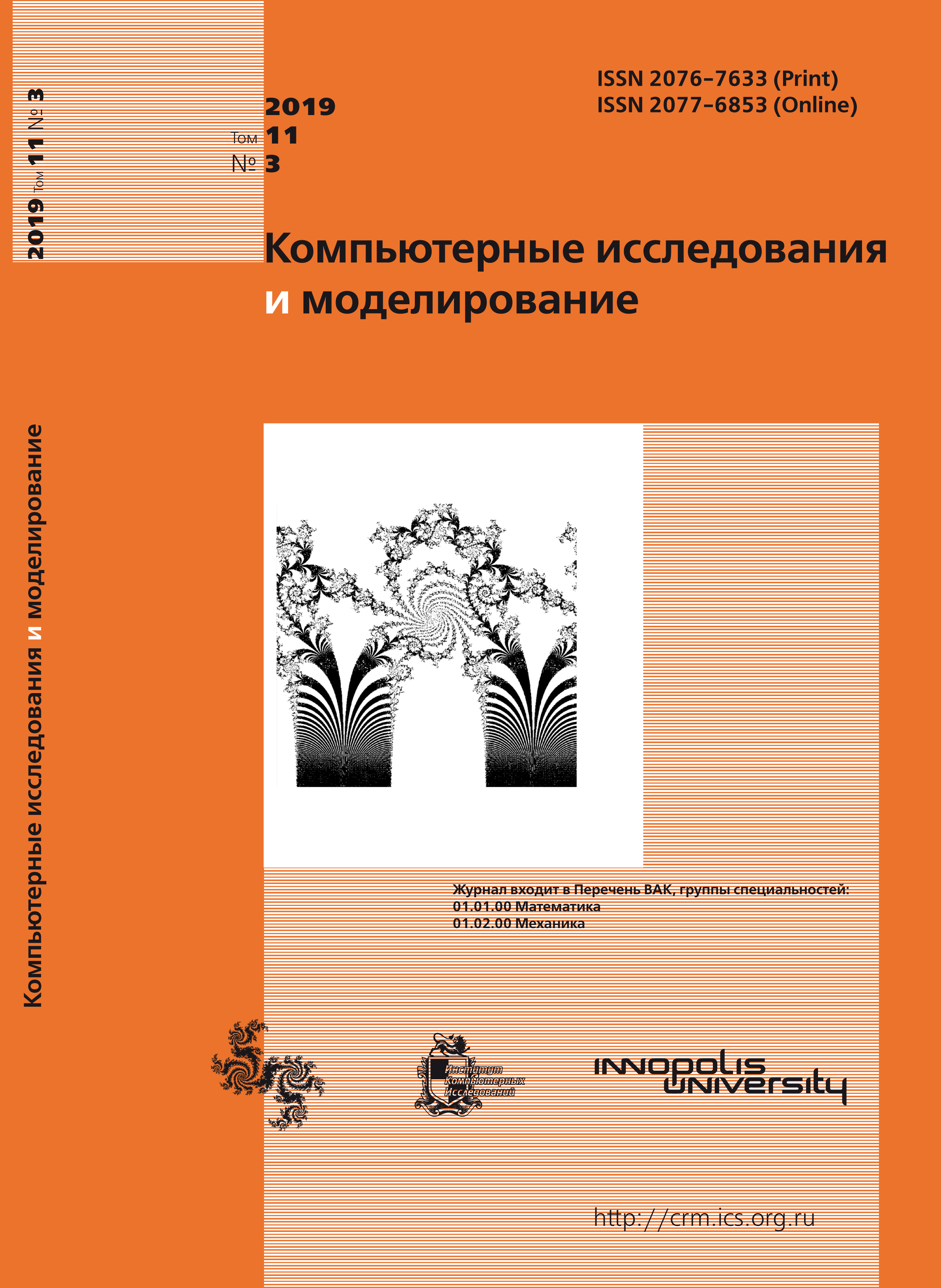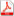Issue 3, 2019 Vol. 11

# All issues

Approximation of analytic functions by repeated de la Vallee Poussin sumspdf (227K)  / List of references

The paper deals with the problems of approximation of periodic functions of high smoothness by arithmetic means of Fourier sums. The simplest and natural example of a linear process of approximation of continuous periodic functions of a real variable is the approximation of these functions by partial sums of the Fourier series. However, the sequences of partial Fourier sums are not uniformly convergent over the entire class of continuous $2\pi$-periodic functions. In connection with this, a significant number of papers is devoted to the study of the approximative properties of other approximation methods, which are generated by certain transformations of the partial sums of Fourier series and allow us to construct sequences of trigonometrical polynomials that would be uniformly convergent for each function $f \in C$. In particular, over the past decades, de la Vallee Poussin sums and Fejer sums have been widely studied. One of the most important directions in this field is the study of the asymptotic behavior of upper bounds of deviations of arithmetic means of Fourier sums on different classes of periodic functions. Methods of investigation of integral representations of deviations of polynomials on the classes of periodic differentiable functions of real variable originated and received its development through the works of S.M. Nikol’sky, S.B. Stechkin, N.P. Korneichuk, V.K. Dzadyk, etc.

The aim of the work systematizes known results related to the approximation of classes of periodic functions of high smoothness by arithmetic means of Fourier sums, and presents new facts obtained for particular cases. In the paper is studied the approximative properties of $r$-repeated de la Vallee Poussin sums on the classes of periodic functions that can be regularly extended into the fixed strip of the complex plane. We obtain asymptotic formulas for upper bounds of the deviations of repeated de la Vallee Poussin sums taken over classes of periodic analytic functions. In certain cases, these formulas give a solution of the corresponding Kolmogorov–Nikolsky problem. We indicate conditions under which the repeated de la Vallee Poussin sums guarantee a better order of approximation than ordinary de la Vallee Poussin sums.

Keywords: Fourier series, Poisson integral, asymptotic formula
Citation in English: Rovenska O.G. Approximation of analytic functions by repeated de la Vallee Poussin sums // Computer Research and Modeling, 2019, vol. 11, no. 3, pp. 367-377
Citation in English: Rovenska O.G. Approximation of analytic functions by repeated de la Vallee Poussin sums // Computer Research and Modeling, 2019, vol. 11, no. 3, pp. 367-377
DOI: 10.20537/2076-7633-2019-11-3-367-377
Views (last year): 45.

Full-text version of the journal is also available on the web site of the scientific electronic library eLIBRARY.RU

The journal is included in the Russian Science Citation Index

The journal is included in the List of Russian peer-reviewed journals publishing the main research results of PhD and doctoral dissertations.

International Interdisciplinary Conference "Mathematics. Computing. Education"

The journal is included in the RSCI

Indexed in Scopus